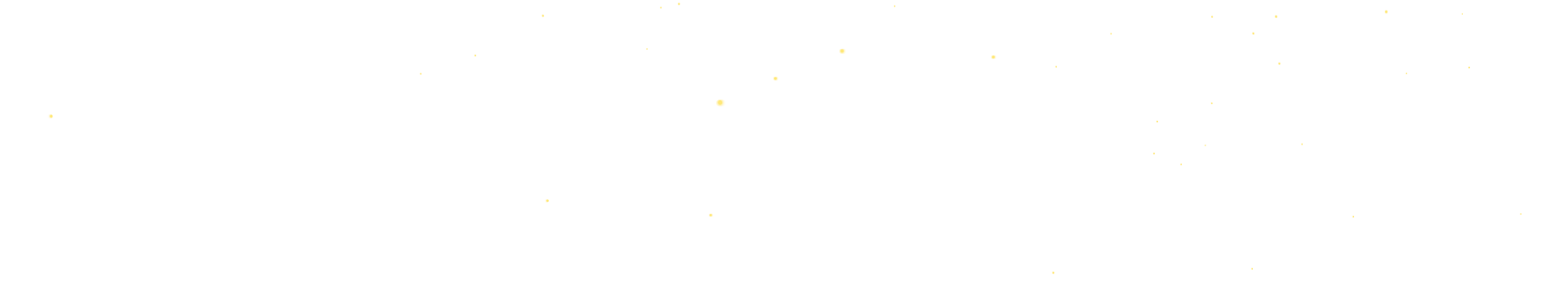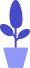# Python turtle module introduction

Estimated reading: 10 minutes 58 views

There is a turtle module in the Python standard library, commonly known as turtle drawing, which provides some simple drawing tools that can draw various graphics in standard application windows.

Turtle’s drawing method is very simple and intuitive, like a small turtle with paint on its tail crawling on a computer screen, and can draw lines as it moves. Using Turtle Drawing, we were able to create impressive visual effects with just a few lines of code, but also follow the turtle’s movement and see how each line of code affects its movement.

This can help us better understand the logic of the code. So turtle drawing is also often used as a tool for beginners to learn Python.

## Create a canvas

Let’s see how turtles work. First, we’re going to import the turtle module. Then we want to create a blank window as a canvas, the size of the window is 800 pixels wide and 800 pixels high. Then create a brush and set the cursor shape to a turtle. code show as below.

``````import turtle
window=turtle.Screen()
turtle.setup(width=800, height=800)
t=turtle.Pen()
turtle.shape("turtle")``````

Running this code, we can see a blank window with a small turtle in the middle, as shown in Figure 1.figure 1
The drawing area of ​​the turtle program window uses a Cartesian coordinate system, and a coordinate system consisting of X and Y coordinates can be used to map the stage to a logical grid.

We set the size of the window to be 800 pixels wide and high. The coordinates of the X axis are from −400 to 400, and the coordinates of the Y axis are also from −400 to 400. The initial position of the turtle is in the center (0,0) of the drawing area of ​​the window, with its head facing the positive direction of the X-axis, as shown in Figure 2.figure 2

Tip: A pixel is a point on the screen, the smallest element that can be represented. Everything we see on the screen is made up of pixels.

## moving turtles

Next, we want the turtle to move. There are many commands to control the turtle’s movement. Let’s first look at a simple command, using the forward() method to move the turtle forward 100 pixels:

``````turtle.forward(100)
``````

forward is the command to move the turtle forward, and 100 is the distance to move. The command to move the turtle backward is backward, and the parameter in parentheses is the distance to move, in pixels. In addition, we can also make the turtle change direction. Command left is to turn left, and command right is to turn right. At this time, the parameter in parentheses indicates the angle to be rotated.

Let’s look at an example, let the turtle draw a square, the code is as follows.

``````import turtle
turtle.forward(100)
turtle.left(90)
turtle.forward(100)
turtle.left(90)
turtle.forward(100)
turtle.left(90)
turtle.forward(100)``````

Running this code, you can see that the turtle has drawn a square, and it is facing down, as shown in Figure 3.image 3
You may be curious, although it is called turtle mapping, but we have not seen any traces of turtles.

This is because, by default, the cursor is an arrow. If you want to see this cute little turtle, you need to call the shape() method and pass “turtle” as a parameter to this method. In addition, you can also call setheading() to set the direction of the little turtle’s movement when it starts. Its parameter is a number, which indicates the angle to be rotated. Let’s look at an example, the code is as follows.

``````import turtle
turtle.shape("turtle")
turtle.forward(100)

In this code, we first change the cursor to a small turtle, then draw a straight line, and then let the small turtle turn around, the final effect is shown in Figure 4.Figure 4
There is also a home() method, which tells the turtle to return to the initial brush position. We add a sentence turtle.home() after the code just now, the code is as follows.

``````import turtle
turtle.shape("turtle")
turtle.forward(100)
turtle.home()``````

Then the final result is that the little turtle has returned to its original position, as shown in Figure 5.Figure 5
In addition to drawing straight lines, baby turtles can also draw circles and arcs. We use the circle() function to draw a circle with a given radius. This function has 3 parameters, namely:

• radius: radius, a positive number means that the center of the drawn circle is on the left side of the brush, and a negative number means that the center of the drawn circle is on the right side of the brush;
• extent: radian, this is an optional parameter, if no value is specified, it means to draw a circle;
• steps: Make the inscribed regular polygon of the circle with radius radius, and the polygon side number is steps. This is also an optional parameter.

Let’s try to draw a circle and see the effect. The code is as follows.

``````import turtle
turtle.circle(100,360)``````

The resulting graph is shown in Figure 6.Image 6
There are two more frequently used methods related to movement:

• turtle.goto(x,y) can position the pen to the specified coordinates;
• turtle.speed(speed) can modify the speed at which the brush runs.

Next, let’s try to draw a kite. First draw a line through the goto() method, representing the kite line, and move the cursor to the upper left corner. Then specify the turtle to run at a speed of 2. Next, draw the head of the kite using the for loop described earlier. code show as below

``````import turtle
turtle.speed(2)
turtle.goto(-200,200)
for x in range(30):
turtle.forward(x)
turtle.left(90)``````

The results obtained are shown in Figure 7.Figure 7

## Brush Control

We can control the start and end of the brush to decide whether to leave a trail of motion on the screen.

• When the penup() method is called, it means that the pen is started, and the trajectory of the movement will not be drawn in this state;
• When the pendown() method is called, it means the pen is down, and the trajectory of the movement will be drawn in this state.

We can understand it this way, the turtle takes a pen, the pen is facing up or down, when the pen is up, the turtle doesn’t draw anything during the movement, and when the pen is down, the turtle draws its own trajectory.

We slightly modified the code for drawing the kite just now. Now, draw 5 similar kite heads in the center of the canvas and the 4 corners of the upper, lower, left and right, and increase the drawing speed to 10. code show as below.

``````import turtle
turtle.speed(10)
for x in range(100):
turtle.forward(x)
turtle.left(90)
turtle.penup()
turtle.goto(-200,200)
turtle.pendown()
for x in range(100):
turtle.forward(x)
turtle.left(90)
turtle.penup()
turtle.goto(200,200)
turtle.pendown()
for x in range(100):
turtle.forward(x)
turtle.left(90)
turtle.penup()
turtle.goto(-200,-200)
turtle.pendown()
for x in range(100):
turtle.forward(x)
turtle.left(90)
turtle.penup()
turtle.goto(200,-200)
turtle.pendown()
for x in range(100):
turtle.forward(x)
turtle.left(90)``````

Running this code, the resulting graph is shown in Figure 8.Figure 8

## color

Turtle drawing is not limited to drawing with a black brush, you can also use other color brushes, and you can even fill the shape with color. Let’s introduce a few functions related to color.

• pencolor: set the brush color;
• fillcolor: set the fill color;
• begin_fill: called before filling the shape;
• end_fill: Called after filling the shape.

Light has 3 main colors: red, green, and blue (red, blue, and yellow are the main colors of painting and paint, but computer monitors use light, not paint). Any other color can be formed by combining different amounts of these 3 colors.

In Pygame, we use tuples of 3 integers to represent colors. The 1st value in the tuple indicating how much red is in the color. An integer value of 0 means that there is no red in the color, and 255 means that the red in the color is at its maximum value. The 2nd value represents green, and the 3rd value represents blue. These are tuples of 3 integers used to represent a color, often called RGB values.

Since we can use any combination of 0 to 255 for the 3 main colors, this means that Pygame can draw 16 777 216 different colors, or 256 x 256 x 256 colors. However, if you try to use a value greater than 255 or a negative value, you will get an error like “ValueError: invalid color argument”.

For example, we create the tuple (0, 0, 0) and store it in a variable called BLACK. Without the amount of red, green and blue color, the final color is completely black. Black is literally nothing of any color value. The tuple (255, 255, 255) represents the maximum amount of red, green, and blue, resulting in white. White is a complete combination of red, green and blue. The tuple (255, 0, 0) represents the maximum amount of red without green and blue, so the final color is red. Similarly, (0, 255, 0) is green and (0, 0, 255) is blue.

Let’s see how to use color with a simple example:

• First, call the pencolor() method to set the brush to red, then call the fillcolor() method to set the fill color to green;
• Then call the begin_fill() method to indicate that filling is to begin;
• Next, call the circle() method to draw a circle, the brush is red, the fill is green, and the radius is 90 pixels;
• Finally, the end_fill() method is called to end the filling.

code show as below.

``````import turtle
turtle.pencolor("red")
turtle.fillcolor("green")
turtle.begin_fill()
turtle.circle(90)
turtle.end_fill()``````

Run it, and the drawn graphic effect is shown in Figure 9.Figure 9
In addition to directly entering the “English name” of the color (such as red, green in the sample code above), we can also directly specify the RGB color value of the color.

Turtle drawing has a special colormode function to specify the RGB color value range is an integer from 0 to 255 or a decimal from 0 to 1. When the parameter is 255, it means that the integer value from 0 to 255 is used; when the parameter is 1.0, it means that the decimal value from 0 to 1 is used. Let’s look at an example, the code is as follows.

``````import turtle
turtle.colormode(255)
turtle.pencolor(255,192,203)
turtle.circle(90)
turtle.colormode(1.0)
turtle.pencolor(0.65,0.16,0.16)
turtle.circle(45)``````

We call the colormode() function twice, the first time the parameter passed to it is 255, and the next call to the pencolor() function uses an integer between 0 and 255 as a parameter to represent the RGB color value, indicating that the color of the brush is Pink. Then draw a big circle.

Next we call the colormode() function again, and the next call to the pencolor() function uses a decimal between 0 and 1 as an argument to represent the RGB color value, indicating that the color of the pen is brown. Then draw a small circle. Run the code, and the result is shown in Figure 10.